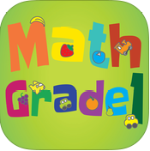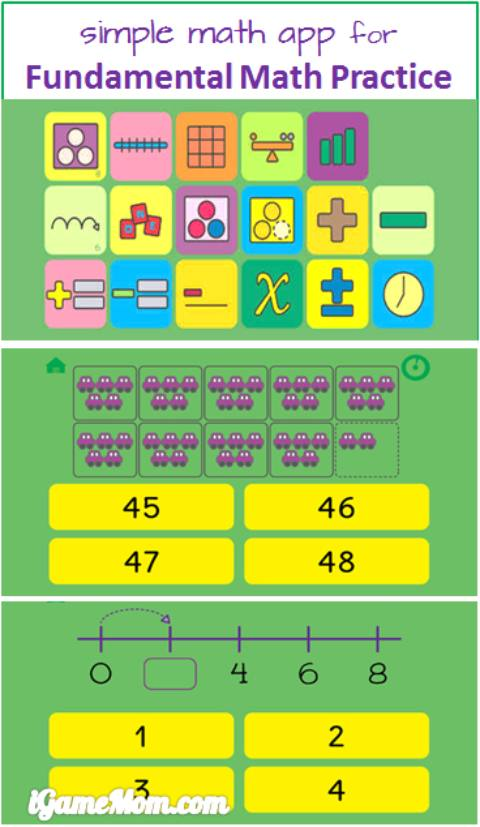≡ Categories

# Practice Math with MathLab AppThere is never too many math practice for kids. The more they practice, the better they are at it. It is especially true for those fundamental math concepts and math facts, such as counting, sequencing, 4 operations within 20. MathLab is an app designed for kids to practice these math concepts and math facts. The design interface is simple, making it very easy to use.There are two sections for practice in the app. Expedition is to going through a 30-step path with progressively more difficult math problems. Laboratory is a collection of 17 labs of math, each lab is for one type of math practice, like number line, telling time.

The 17 labs in the Laboratory sections are:
Counting: kids see pictures showing number of objects and choose the correct number corresponding to the picture. For bigger numbers kids will see objects grouped in ten’s.

Number Lines: kids fill in the missing number on the number line. It is a way to learn orders.

Hundred number chart: kids pick the right number for the missing spot on the hundred number chart.

Odd Even number: kids see number of objects and answer if it is an odd or even number.

Order: kids are given several number, and are asked to put them in order from the smallest to the biggest.

Skip counting using a number line: kids fill in the missing number on the number line. All neighboring numbers have a fix difference of more than 1, like 2, 5, or 10.

Name the number: kids are shown a numeric number and are asked to pick the right name of the number in English.

Addition: Kids are shown pictures of two types of objects, and are asked to add the number of each type together. They are all additions within 10.

Subtraction: different from Addition, kids will see pictures of objects with some being crossed out. Kids need find out how many left.

Addition with numbers: This is a more advanced addition. All problems are shown with actual numbers, not pictures. The problems are within 20.

Subtraction with numbers: subtraction problems with actual numbers within 20.

Reversed addition: kids are given the result number, and are asked to pick the equation that will lead to the answer. For example, for the number 10, they should choose 3+2.

Reversed subtraction: similar to Reversed Addition, but this is for subtraction.

Simple Algebra: kids won’t see x or y in the problems, but is to fill in the blank in an equation, like Blank+5=9, and kids need answer which number should go in the blank spot.

Mixed operation: kids will see two operations in one equation, and they are addition or subtraction. All numbers are within 10.

Time: kids are show a clock and are asked to choose the correct time shown on the picture.

Overall it is a good math practice math with very good coverage for those just started math. Depends on your child’s level, a child from preschool to first grade will find this app helpful. It is available on iPhone, iPod and iPad. It is \$1.99.

If you would like to check it out or purchase it, please use the App Store link provided below. The cost is the same to you, but iGameMom gets a small percentage. Thanks for your support! Note: The link works for all countries.